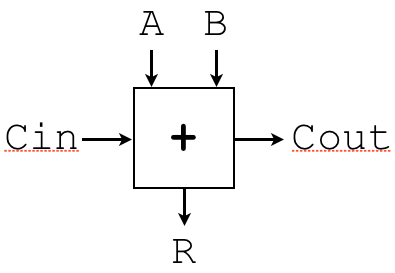# Lab 8: K-Maps with Don't Cares, Multiplexers, and ALUs

Due Thursday, August 25 at 11:59 PM

## Goals for This Lab

By the time you have completed this work, you should be able to:

• Simplify truth tables containing don't cares with K-maps
• Build multiplexers of various sizes ultimately from `AND`, `OR`, and `NOT` gates
• Build simple ALUs ultimately from `AND`, `OR`, and `NOT` gates
Provided files:

## Step 1: Edit the `lab8problems.txt` File

Using a text editor of your choice, open the `lab8problems.txt` file. The file contains a series of questions for you to answer. The answers should be placed directly in the file itself, except where noted. All questions must be answered correctly for full credit. Make sure you save your answers before you exit.

## Step 2: Build a Two-Bit ALU

The last question in `lab8problems.txt` required you to write out a circuit for part of a single-bit adder (that is, a circuit that adds two individual bits together). In this part, you will build a 2-bit ALU (that is, each operand is two bits large) with support for addition and subtraction. To this end, you are given the component below:The above component takes the following single-bit inputs:

• `A`: The first operand
• `B`: The second operand
• `Cin`: The carry-in bit

Given the above inputs, the circuit produces the following single-bit outputs:

• `Cout`: The carry-out bit
• `R`: The result bit

In making your 2-bit ALU, you may use as many of the above components as you want, along with any number of `AND`, `OR`, `NOT`, and 2-input `MUX`s. Overall, your 2-bit ALU takes the following single-bit inputs:

• `Op`: Which operation is to be performed, `0` for addition and `1` for subtraction (`A - B`)
• `A0`: Bit 0 of operand `A`
• `A1`: Bit 1 of operand `A`
• `B0`: Bit 0 of operand `B`
• `B1`: Bit 1 of operand `B`

Given the above inputs, your ALU must produce the following outputs:

• `Cout`: The carry-out bit
• `R0`: Bit 0 of the result
• `R1`: Bit 1 of the result

You must draw your circuit, and submit it as “`lab8alu.jpg`”. You may find the following hints helpful as part of this process:

1. While not strictly required, 2-input `MUX`s will simplify things.
2. To add with multiple bits, you will need to chain multiple “`+`” components together.
3. Recall that you can effectively subtract via addition by flipping all the bits of the second operand, and then adding one. For this addition of one, the initial carry-in bit can be set to `1`.
4. There are two general approaches to implement this, and either will work:
1. Do both the addition and subtraction in parallel, then use a multiplexer to select between the results. This requires a 2-input `MUX` for each output bit. This is straightforward, but it requires a lot of components.
2. Adjust the inputs to the “`+`” component, based on the value of `Op`. This requires more thinking about exactly what values are fed to the “`+`” components, but the resulting circuit is much smaller.

• `lab8problems.txt`
• `lab8smallmux.jpg`
• `lab8bigmux.jpg`
• `lab8cout.jpg`
• `lab8alu.jpg`
• `collaborators.txt`, if you're working with anyone else. Each person you're working with should be listed in the file, with one person per line.